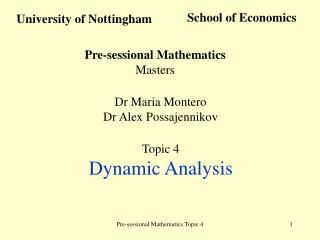DownloadDownload PresentationUniversity of Nottingham

# University of Nottingham

Télécharger la présentation## University of Nottingham

- - - - - - - - - - - - - - - - - - - - - - - - - - - E N D - - - - - - - - - - - - - - - - - - - - - - - - - - -
##### Presentation Transcript

1. School of Economics University of Nottingham Pre-sessional Mathematics Masters Dr Maria Montero Dr Alex Possajennikov Topic 4 Dynamic Analysis Pre-sessional Mathematics Topic 4

2. Dynamic Analysis Modelling dependence of variables on time In economics: growth models, overlapping generations, life-cycle consumption Difference equations Discrete time xt+1 = f(xt , t) xt R Initial condition x0 Solution: xt(x0) Autonomous equations xt+1 = f(xt) Pre-sessional Mathematics Topic 4

3. xt+1 = xt f(x) x Dynamic Analysis Linear difference equation xt+1 = axt + b x= f(x) stationary state Non-linear equation xt+1= f(xt) Phase diagram Suppose x0is stationary If |f’(x0)| < 1 then stable If |f’(x0)| > 1 then unstable x0 Pre-sessional Mathematics Topic 4

4. Dynamic Analysis Differential equations continuous time Ordinary differential equation (ODE) of first order General solution is a function x(t) that satisfies the equation Particular solution is a general solution that satisfies initial conditionx(t0) = x0 Theorem F is continuous at t0, x0. Then there exists unique particular solution x(t) C1 of the equation with x(t0) = x0 Pre-sessional Mathematics Topic 4

5. Dynamic Analysis Solving by integration Autonomous equation Example Linear equation Pre-sessional Mathematics Topic 4

6. F(x) x Dynamic Analysis Stability When F(x0) = 0, xdoes not change x0is stationary point When F(x) > 0, xincreases When F(x) < 0, xdecreases Linear equation Non-linear equation Phase diagram Stationary point x0is asymptotically stable, if any solution with initial conditions close to x0converges to x0 If F’(x0) < 0, then x0 is asymptotically stable If F’(x0) > 0, then x0 is unstable Pre-sessional Mathematics Topic 4

7. Dynamic Analysis Systems of differential equations Initial condition (x10,…,xn0,t0) If functions are continuous, solution x1(t),…,xn(t)exists and unique Linear systems (autonomous) Suppose that A is diagonal Solution: Suppose that Adiagonalisable P-1AP = D Transformation of variables z = P-1x x = P z Pre-sessional Mathematics Topic 4

8. Theorem Solution of where iare eigenvalues of A, vi are eigenvectors of A Dynamic Analysis Example Initial conditions x(0) = -1, y(0) = 2 Pre-sessional Mathematics Topic 4

9. Dynamic Analysis Stability Point x0 =(x10,…,xn0) is stationary state (steady state, equilibrium) if fi(x10,…,xn0) = 0 for all i. Linear systems Point x0 =(0,…,0) is stationary state Stationary point x0is asymptotically stable, if any solution with initial conditions close to x0converges to x0 If i > 0 for somei, then x(t)  unstable If i < 0 for alli, then x(t)  0 stable Pre-sessional Mathematics Topic 4

10. Dynamic Analysis x0 =(x10,…,xn0) is stationary state Jacobian of the system Theorem If alleigenvalues of the Jacobian are negative, then x0is asymptotically stable If some eigenvalues are positive, then x0is unstable Pre-sessional Mathematics Topic 4

11. Dynamic Analysis Example Pre-sessional Mathematics Topic 4

12. y x Dynamic Analysis Phase diagram (vector field) Example When f > 0, x is increasing When f < 0, x is decreasing When g > 0, y is increasing When g < 0, y is decreasing Pre-sessional Mathematics Topic 4

13. Dynamic Analysis Linear systems of difference equations x1t+1 = a11x1t +…+a1nxnt … xnt+1 = an1x1t +…+annxnt xt+1 = Axt P-1AP = D Transformation of variables z = P-1x x = P z Theorem Solution of xt+1 = Axt is xt = c1(x0)·(1)tv1 +…+ cn (x0)·(n)tvn where iare eigenvalues of A, vi are eigenvectors of A xt+1= Axt + b Pre-sessional Mathematics Topic 4

14. Dynamic Analysis Example xt+1 = 3xt +2yt yt+1 = 2xt Stability xt+1 = Axt x= Axstationary (steady) state x= 0is a stationary state xt = c1(x0)·(1)tv1 +…+ cn (x0)·(n)tvn If |i| > 1 for somei, then xt If |i| < 1 for alli, then xt  0 unstable stable Pre-sessional Mathematics Topic 4

15. Dynamic Analysis Optimisation over time Discrete time maxt=0Tf(ct,kt,t) s.t.kt+1 - kt = g(ct,kt,t) ct is control variable k0 = K0 kt is state variable Lagrangian L = t=0Tf(ct,kt,t) + t(g(ct,kt,t)) - (kt+1 - kt)) + (k0 - K0) L/ct = fc(ct,kt,t) +t gc(ct,kt,t) = 0 t = 1,…,T L/kt = fk(ct,kt,t) +t gk(ct,kt,t) + (t - t-1)= 0 t = 1,…,T Since t is shadow price, -(t - t-1) is the rate of depreciation of k On optimal path, the rate of depreciation of kequals its contribution to f and to g Hamiltonian H(ct,kt,t,t) = f(ct,kt,t) +t g(ct,kt,t) Pre-sessional Mathematics Topic 4

16. Dynamic Analysis Continuous time Fixed endpoint k(0)= K0, k(T) = KT Solution: functionsc*(t),k*(t) Hamiltonian H(c,k,,t) = f(c,k,t) +g(c,k,t) Suppose functions f,g are differentiable Theorem On the interior optimal path c*(t),k*(t) k(0)= K0, k(T) = KT Pre-sessional Mathematics Topic 4

17. Dynamic Analysis Example k(0)= 4, k(10) = 24 Pre-sessional Mathematics Topic 4

18. Dynamic Analysis Free endpoint k(0)= K0 Hamiltonian k(0)= K0 (T)= 0 Transversality condition Pre-sessional Mathematics Topic 4

19. Dynamic Analysis Example k(0)= 3 Pre-sessional Mathematics Topic 4

20. Dynamic Analysis Discounting k(0)= K0 Hamiltonian H(c,k,,t) = f(c,k)e-t +g(c,k) Current value Hamiltonian H0 = Het = f(c,k) +g(c,k) Pre-sessional Mathematics Topic 4

21. Dynamic Analysis k(0)= K0 Current value Hamiltonian H0 = f(c,k) +g(c,k) k(0)= K0 (T)= 0 Pre-sessional Mathematics Topic 4

22. Dynamic Analysis Example k(0)= K0 Pre-sessional Mathematics Topic 4

23. Dynamic Analysis Infinite horizon k(0)= K0 Current value Hamiltonian H0 = f(c,k) +g(c,k) k(0)= K0 Transversality condition When the system of differential equations has a saddle point equilibrium with k0 , use Pre-sessional Mathematics Topic 4

24. Dynamic Analysis Example k(0)= K0 Pre-sessional Mathematics Topic 4